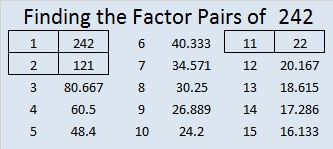# 242 and Level 4

• 242 is a composite number.
• Prime factorization: 242 = 2 x 11 x 11, which can be written 2 x (11^2)
• The exponents in the prime factorization are 1 and 2. Adding one to each and multiplying we get (1 + 1)(2 + 1) = 2 x 3 = 6. Therefore 242 has 6 factors.
• Factors of 242: 1, 2, 11, 22, 121, 242
• Factor pairs: 242 = 1 x 242, 2 x 121, or 11 x 22
• Taking the factor pair with the largest square number factor, we get √242 = (√2)(√121) = 11√2 ≈ 15.556Print the puzzles or type the factors on this excel file: 12 Factors 2014-09-22This site uses Akismet to reduce spam. Learn how your comment data is processed.